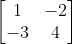## Matrix Inverse

What is the inverse of the below matrix?Hint
The inverse of a square $$n\times n$$ matrix is:
$$A^{-1}=\frac{adj(A)}{|A|}$$$where $$|A|$$ is the determinant of matrix A, and $$adj(A)$$ is the adjoint of matrix A, which is obtained by replacing $$A^T$$ elements with their cofactors. Hint 2 $$\begin{bmatrix}a & b\\ c & d\end{bmatrix}^{-1}=\frac{1}{ad-bc}\begin{bmatrix}d & -b\\ -c & a\end{bmatrix}$$$
The inverse of a square $$n\times n$$ matrix is:
$$A^{-1}=\frac{adj(A)}{|A|}$$$where $$|A|$$ is the determinant of matrix A, and $$adj(A)$$ is the adjoint of matrix A, which is obtained by replacing $$A^T$$ elements with their cofactors. Thus, for a 2 x 2 matrix: $$\begin{bmatrix}a & b\\ c & d\end{bmatrix}^{-1}=\frac{1}{ad-bc}\begin{bmatrix}d & -b\\ -c & a\end{bmatrix}$$$
Therefore,
$$\begin{bmatrix}1 & -2\\ -3 & 4\end{bmatrix}^{-1}=\frac{1}{(1)(4)-(-2)(-3)}\begin{bmatrix}4 & 2\\ 3 & 1\end{bmatrix}$$$$$=\frac{1}{4-6}\begin{bmatrix}4 & 2\\ 3 & 1\end{bmatrix}=-\frac{1}{2}\begin{bmatrix}4 & 2\\ 3 & 1\end{bmatrix}=\begin{bmatrix}-2 & -1\\ -1.5 & -0.5\end{bmatrix}$$$
Note: we can check our work by multiplying the original matrix with its inverse. The resultant is the identity matrix ( $$n\times n$$ matrix with 1’s on the diagonal and 0’s everywhere else).
$$[A][A]^{-1}=[A]^{-1}[A]=[I]$$$where $$[I]$$ is the identity matrix, $$[A]$$ is the original matrix, and $$[A]^{-1}$$ is the inverse matrix. $$\begin{bmatrix}-2 & -1\\ -1.5 & -0.5\end{bmatrix}$$$